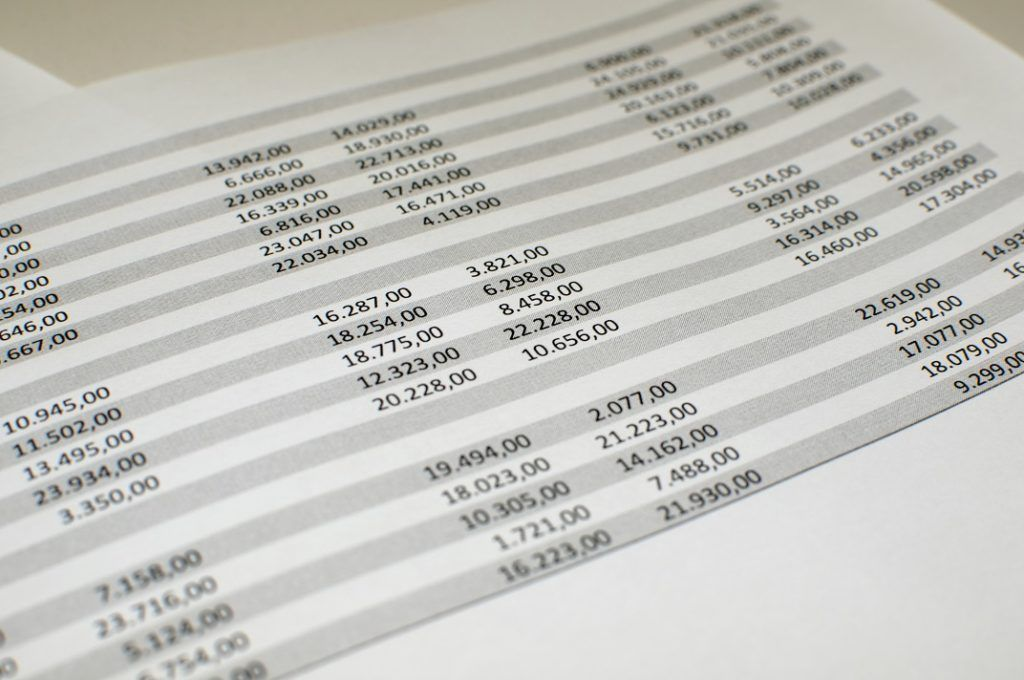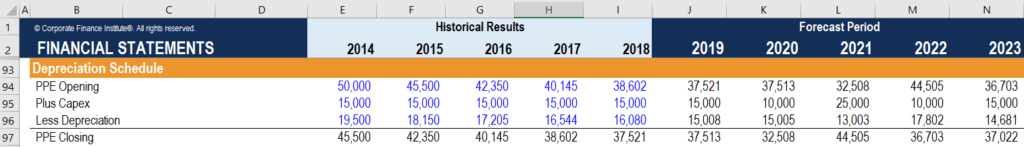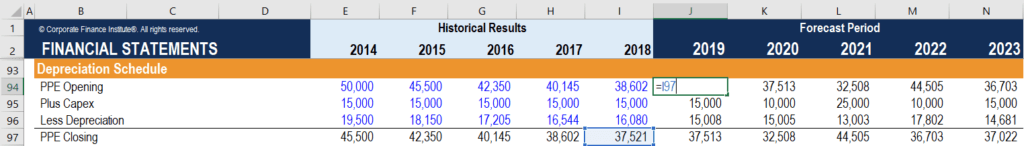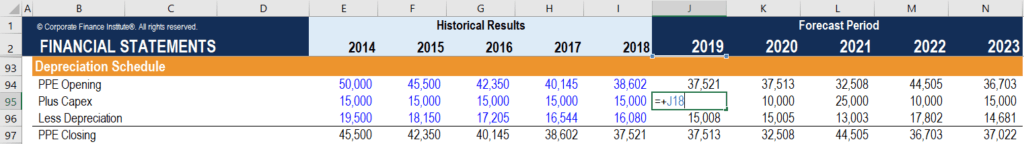# Corkscrew Calculation

The ending balance from the previous period is used as the beginning balance in the current period

## What is Corkscrew Calculation?

In financial modeling, corkscrew calculation (also known as roll-forward calculation or corkscrew accounts) allows the consistent modeling of balances. Essentially, in a corkscrew calculation, the ending balance from the previous period is used as the beginning balance in the current period (in other words, the balance is rolled forward).### Components of Corkscrew Calculation

Every corkscrew calculation consists of the following four components:

#### 1. Beginning balance

The beginning balance in the current period is always equal to the ending balance in the previous period.

#### 2. Upward flow

A flow that increases the beginning balance. For instance, in PP&E calculation, capital expenditures (CapEx) are upward flows as they are added to the beginning PP&E balance.

#### 3. Downward flow

A flow that decreases the beginning balance. For example, in PP&E calculation, depreciation is a downward flow because it is subtracted from the beginning PP&E balance.

#### 4. Ending balance

The ending balance is calculated using the three previous components. All upward flows are added to the beginning balance while all downward flows are subtracted from the beginning balance. Then, the ending balance of the current period is rolled forward, and it is used as the beginning balance for the next period.

Corkscrew calculations are an essential component of detailed and informative financial models. The calculations ensure the consistency and integrity of a model. In addition, they provide the reader of the model with a simple approach to absorb the included information.

In financial models, corkscrew accounts are primarily used in various supporting schedules such as the PP&E schedule, accounts payable schedules, and retained earnings balances.

### How to Use Corkscrew Calculation in Excel?

Since Microsoft Excel is a primary tool in financial modeling, one should know how to incorporate corkscrew calculations in a financial model created in Excel.

We will discover how to create a corkscrew calculation in Excel by following the steps necessary to develop the depreciation schedule:

1. Identify the four components of the corkscrew calculation. In our case, the components include PPE Opening Balance, CapEx (upward flow), Depreciation (downward flow), and PPE Closing Balance.
2. List all the components in one column. For each upward and downward flow, use words Plus and Less to indicate whether the flow is added to or subtracted from the opening balance. In such a case, the reader of the model will clearly see the logic of the calculations of the Closing Balance.3. For the historical periods, use hardcoded values for Opening Balance, Upward Flows, and Downward Flows. The Closing Balance should be calculated using a formula.
4. Remember that the Opening Balance in the current period is essentially the Closing Balance from the previous period. Thus, each Opening Balance in the forecasted periods must be referenced to the Closing Balance from the previous period. In the picture below, the Opening Balance for 2019 is the reference to the Closing Balance for 2018.5. Do not hardcode the Upward and Downward Flows (CapEx and Depreciation in our example) in the forecasted periods. Use the references to the assumptions as shown in the image below.6. The Closing Balances should be calculated using a formula.

CFI offers the Financial Modeling & Valuation Analyst (FMVA)™ certification program for those looking to take their careers to the next level. To keep learning and advancing your career, the following CFI resources will be helpful:

• Financial Modeling Best Practices
• Depreciation Methods
• PP&E (Property, Plant and Equipment)
• Retained Earnings

### Free Excel Tutorial

To master the art of Excel, check out CFI’s FREE Excel Crash Course, which teaches you how to become an Excel power user.  Learn the most important formulas, functions, and shortcuts to become confident in your financial analysis.

Launch CFI’s Free Excel Course now to take your career to the next level and move up the ladder!